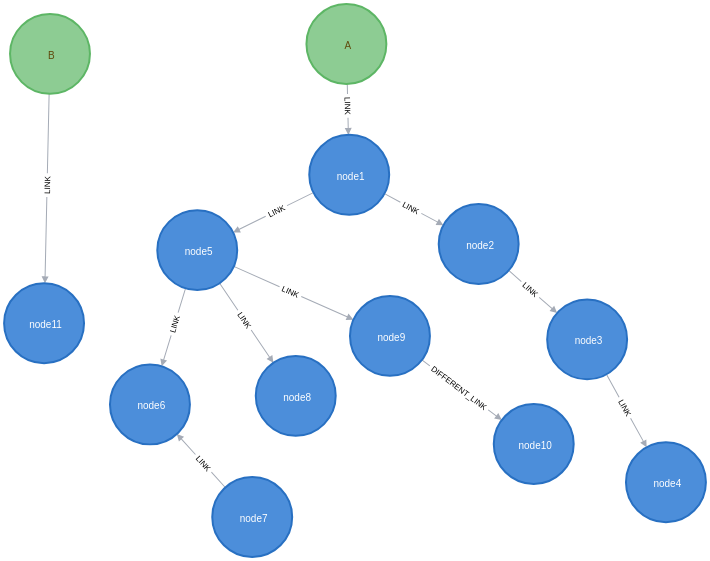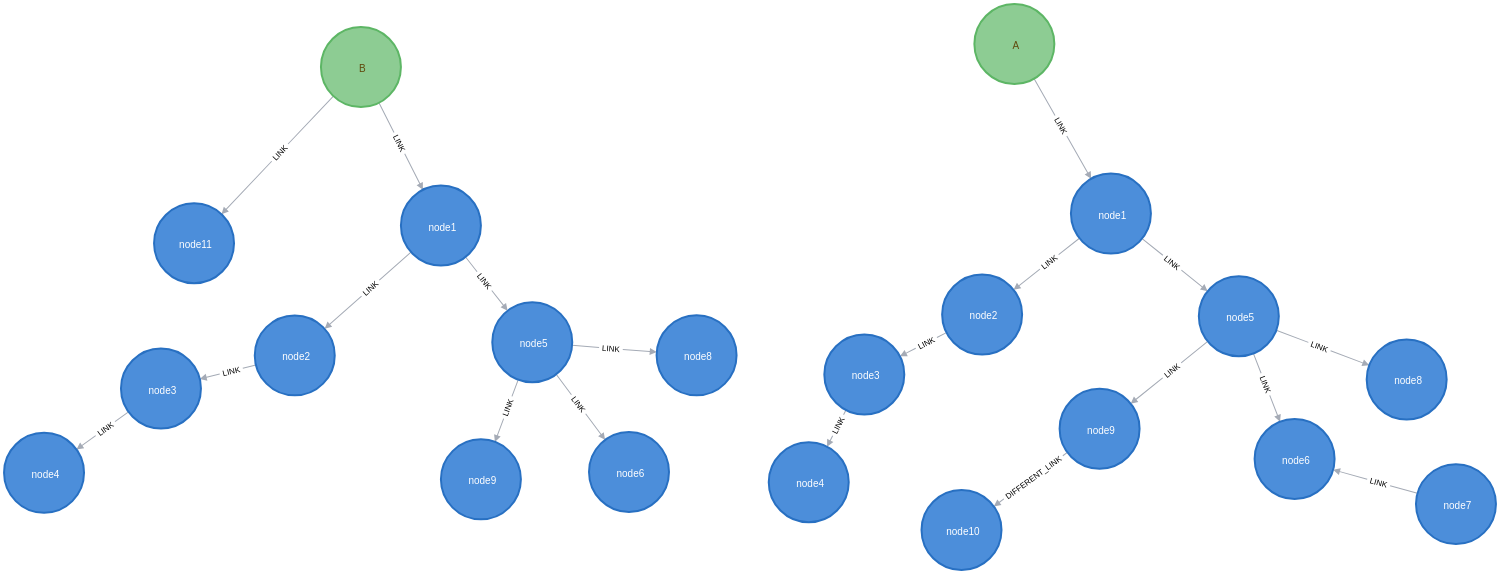# Clone subgraph

These procedures can be used to clone a subgraph defined either by a list of nodes and a list of relationships, or a list of paths. This is useful when you want to ensure the cloned subgraph isn’t connected to the original nodes, or to nodes outside the subgraph.

If relationships are not provided, all relationships between the given nodes will be cloned.

In the config map, we can supply a `standinNodes` list (of pairs of nodes), allowing an existing node in the graph to act as a standin for another node in the cloned subgraph. This can be useful when you want to attach the cloned subgraph to another node in your graph (in place of cloning a node).

The available procedures are described in the table below:

Qualified Name Type Release

`Procedure`

`APOC Core`

`Procedure`

`APOC Core`

## Example Usage

The examples below will help us learn how to use these procedures.

### Cloning a tree from one root to another

The following creates a dataset containing two trees:
``````CREATE  (rootA:Root{name:'A'}),
(rootB:Root{name:'B'}),
(n1:Node{name:'node1', id:1}),
(n2:Node{name:'node2', id:2}),
(n3:Node{name:'node3', id:3}),
(n4:Node{name:'node4', id:4}),
(n5:Node{name:'node5', id:5}),
(n6:Node{name:'node6', id:6}),
(n7:Node{name:'node7', id:7}),
(n8:Node{name:'node8', id:8}),
(n9:Node{name:'node9', id:9}),
(n10:Node{name:'node10', id:10}),
(n11:Node{name:'node11', id:11}),
(n12:Node{name:'node12', id:12})
CREATE (rootA)-[:LINK]->(n1)-[:LINK]->(n2)-[:LINK]->(n3)-[:LINK]->(n4)
CREATE                  (n1)-[:LINK]->(n5)-[:LINK]->(n6)<-[:LINK]-(n7)
CREATE                                (n5)-[:LINK]->(n8)
CREATE                                (n5)-[:LINK]->(n9)-[:DIFFERENT_LINK]->(n10)
CREATE (rootB)-[:LINK]->(n11)``````The following query clones a subtree starting from rootA consisting of outgoing `:LINK` relationships, and attaches that subgraph to rootB. rootB acts as a standin for rootA, which is not cloned:
``````MATCH  (rootA:Root{name:'A'}),
(rootB:Root{name:'B'})
MATCH path = (rootA)-[:LINK*]->(node)
WITH rootA, rootB, collect(path) as paths
CALL apoc.refactor.cloneSubgraphFromPaths(paths, {
standinNodes:[[rootA, rootB]]
})
YIELD input, output, error
RETURN input, output, error``````

If we execute this query, it will result in the following graph:Another approach is to use `apoc.refactor.cloneSubgraph()`, providing the lists of nodes and relationships which form the subgraph. We can get the nodes and rels from the yielded output of `apoc.path.subgraphAll()`, filtering to the relationship types in the call to that procedure.

The following query creates a dataset containing two trees:
``````CREATE  (rootA:Root2{name:'A'}),
(rootB:Root2{name:'B'}),
(n1:Node2{name:'node1', id:1}),
(n2:Node2{name:'node2', id:2}),
(n3:Node2{name:'node3', id:3}),
(n4:Node2{name:'node4', id:4}),
(n5:Node2{name:'node5', id:5}),
(n6:Node2{name:'node6', id:6}),
(n7:Node2{name:'node7', id:7}),
(n8:Node2{name:'node8', id:8}),
(n9:Node2{name:'node9', id:9}),
(n10:Node2{name:'node10', id:10}),
(n11:Node2{name:'node11', id:11}),
(n12:Node2{name:'node12', id:12})
CREATE (rootA)-[:LINK]->(n1)-[:LINK]->(n2)-[:LINK]->(n3)-[:LINK]->(n4)
CREATE                  (n1)-[:LINK]->(n5)-[:LINK]->(n6)<-[:LINK]-(n7)
CREATE                                (n5)-[:LINK]->(n8)
CREATE                                (n5)-[:LINK]->(n9)-[:DIFFERENT_LINK]->(n10)
CREATE (rootB)-[:LINK]->(n11)``````
The following query clones a subtree starting from rootA consisting of outgoing `:LINK` relationships, and attaches that subgraph to rootB. rootB acts as a standin for rootA, which is not cloned:
``````MATCH  (rootA:Root2{name:'A'}),
(rootB:Root2{name:'B'})
CALL apoc.path.subgraphAll(rootA, {relationshipFilter:'LINK>'})
YIELD nodes, relationships
CALL apoc.refactor.cloneSubgraph(
nodes,
[rel in relationships WHERE type(rel) = 'LINK'],
{ standinNodes:[[rootA, rootB]] })
YIELD input, output, error
RETURN input, output, error``````

The resulting graph will be the same as our earlier `apoc.refactor.cloneSubgraphFromPaths()` call.